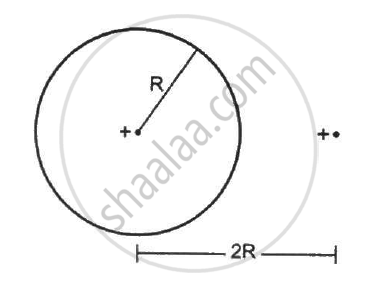Department of Pre-University Education, KarnatakaPUC Karnataka Science Class 12
Share

# Find the Flux of the Electric Field Through a Spherical Surface of Radius R Due to a Charge of 10−7 C at the Centre and Another Equal Charge at a Point 2r Away from the Centre in the Following Figure. - Physics

ConceptUniformly Charged Infinite Plane Sheet and Uniformly Charged Thin Spherical Shell (Field Inside and Outside)

#### Question

Find the flux of the electric field through a spherical surface of radius R due to a charge of 10−7 C at the centre and another equal charge at a point 2R away from the centre in the following figure.#### Solution

Given:-

Let charge Q be placed at the centre of the sphere and Q' be placed at a distance 2R from the centre.

Magnitude of the two charges  = 10−7 C

According to Gauss's Law, the net flux through the given sphere is only due to charge Qthat is enclosed by it and not by the charge Q' that is lying outside.

So, only the charge located inside the sphere will contribute to the flux passing through the sphere.

Thus,

phi = ∫  vec"E" . vec("d"."s") = "Q"/∈_0 = 10^-7/( 8.85 xx 10^-12)

=> phi = 1.1 xx 10^4   "N""m"^2   "C"^-1

Is there an error in this question or solution?

#### Video TutorialsVIEW ALL 

Solution Find the Flux of the Electric Field Through a Spherical Surface of Radius R Due to a Charge of 10−7 C at the Centre and Another Equal Charge at a Point 2r Away from the Centre in the Following Figure. Concept: Uniformly Charged Infinite Plane Sheet and Uniformly Charged Thin Spherical Shell (Field Inside and Outside).
S